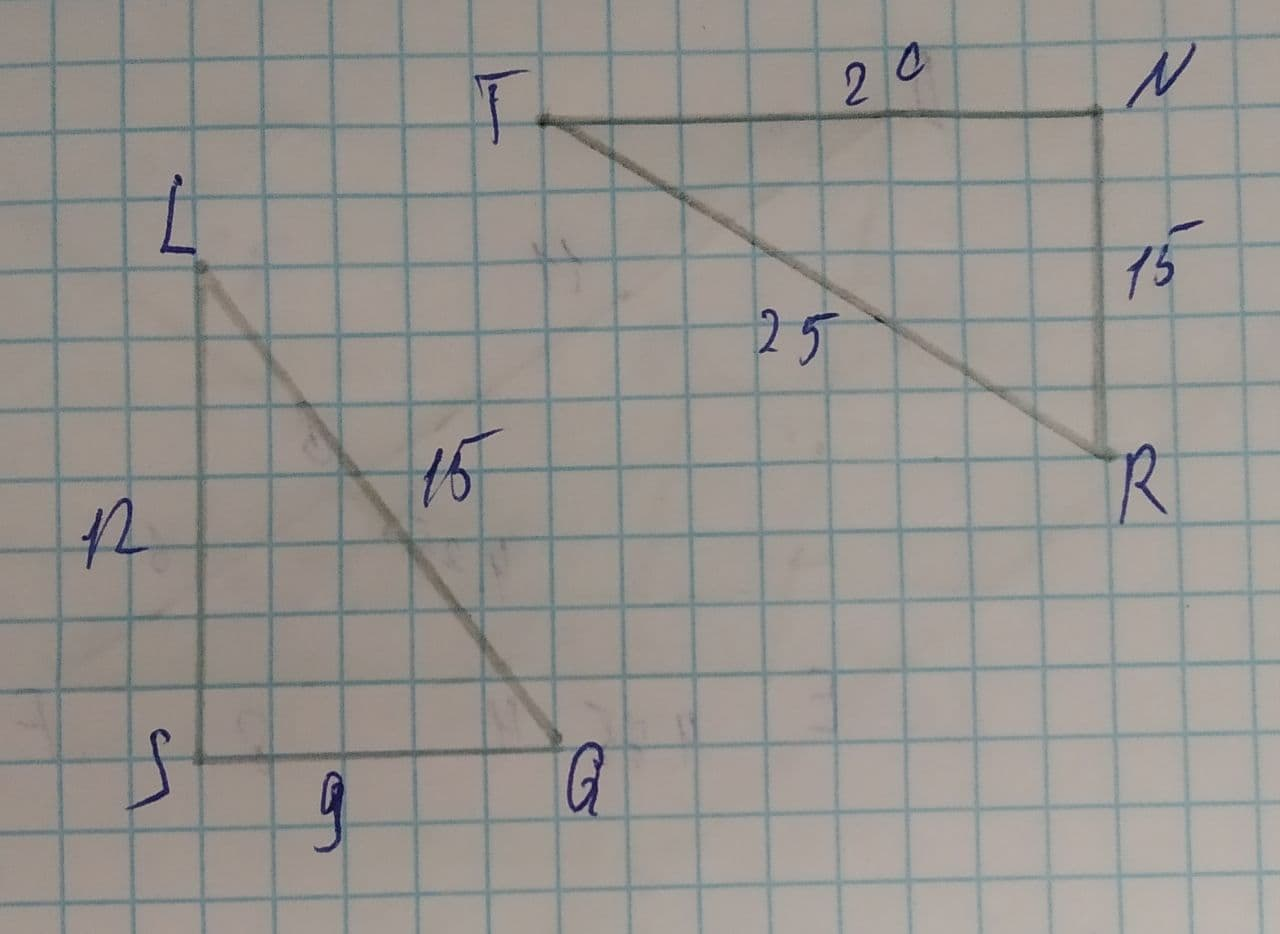# Determine whether the triangles are similar. If so, wrote the similarity statement and name the postulate or theorem you used.Phoebe 2021-08-11 Answered
Determine whether the triangles are similar. If so, wrote the similarity statement and name the postulate or theorem you used.• Questions are typically answered in as fast as 30 minutes

### Plainmath recommends

• Get a detailed answer even on the hardest topics.
• Ask an expert for a step-by-step guidance to learn to do it yourself.dieseisB
Here, $$\displaystyle{\frac{{{L}{Q}}}{{{T}{R}}}}={\frac{{{L}{S}}}{{{T}{N}}}}={\frac{{{S}{Q}}}{{{N}{R}}}}$$
Hence, $$\displaystyle{L}{Q}\sim{T}{R}$$
$$\displaystyle{T}{N}\sim{L}{S}$$
$$\displaystyle{S}{Q}\sim{N}{R}$$
Now, by Side-Side-Side (SSS-rule) similarity both given triangles are similar.